# Solution May 30, 2007

### Problem

Let a,b,c,d be positive reals such that a + b + c + d = 1.

Prove that: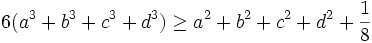$6(a^{3}+b^{3}+c^{3}+d^{3})\geq a^{2}+b^{2}+c^{2}+d^{2}+\frac{1}{8}$.

### Solution

Let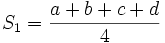$S_1=\frac{a+b+c+d}4$,$S_2=\frac{a^2+b^2+c^2+d^2}4$ and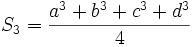$S_3=\frac{a^3+b^3+c^3+d^3}4$. By the power mean inequality (http://planetmath.org/encyclopedia/GeneralMeansInequality.html), we have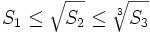$S_1 \leq \sqrt{S_2} \leq \sqrt{S_3}$.

This implies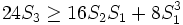$24 S_3 \geq 16 S_2 S_1 + 8 S_1^3$

Using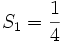$S_1 = \frac 14$, this is equivalent to$6(a^{3}+b^{3}+c^{3}+d^{3})\geq a^{2}+b^{2}+c^{2}+d^{2}+\frac{1}{8}$

q.e.d.